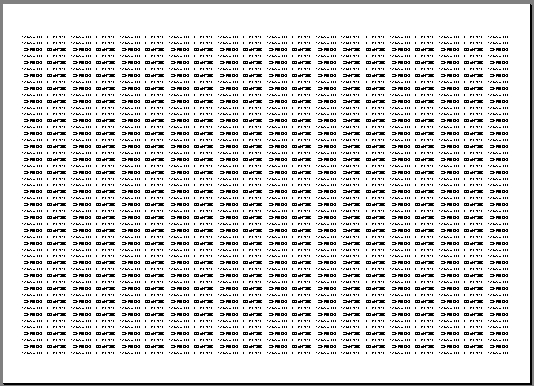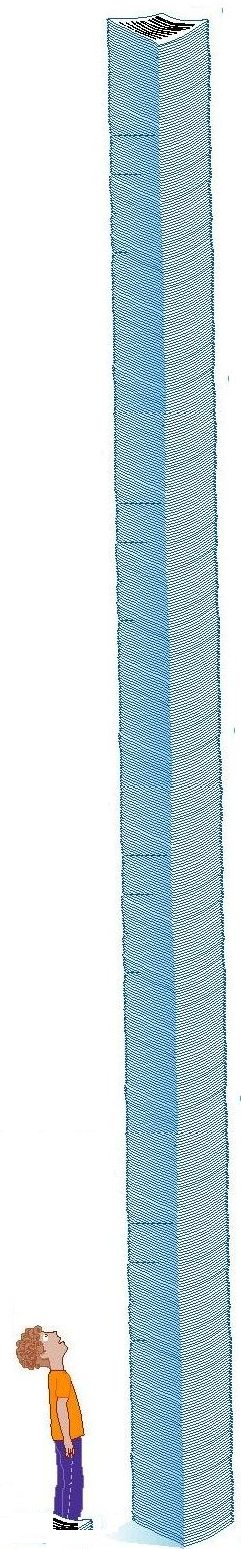#Million Phone Numbers

## How many is that?

If you printed themillion numbers out, 1000 numbers per page, that would be 50 rows by 20 columns

This is a small font but is still readable.

If you stacked those sheets of paper 100 per inch, standard for a ream of paper, it would create a stack of

107,000,000 numbers divided by 1000 number per page = 107,000 sheets of paper

107,000 sheets of paper divided by 100 papers per inch = 1070 inches

1070 inches divided by 12 inches per foot = 89 feet high

With a stack of papers 89 feet high, how can you possibly match the numbers with your existing phone databases?1000 phone numbers per page would be 50 rows by 20 columns This is about as many as would be readable per page.